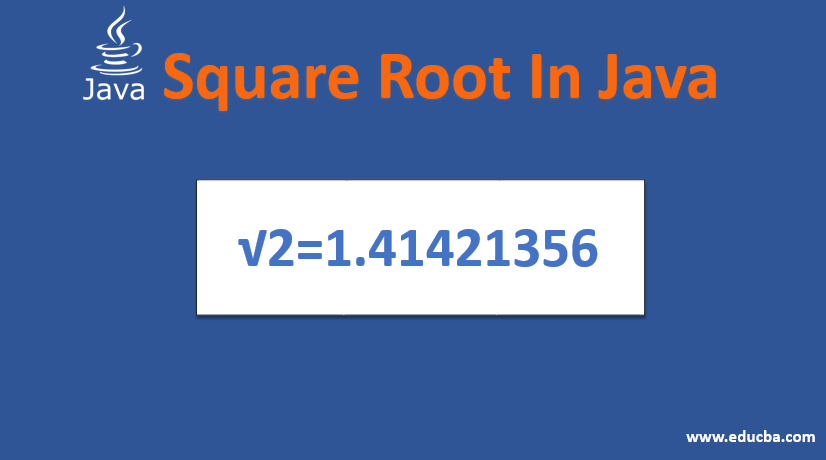## Introduction to Square Root in Java

The Square root of a number can be calculated in Java using sqrt() method from Math class as Math.sqrt() from the Java library. There are several ways to find the square root of a number. The standard or normal method to find the square root of a number is the long division method. This method is difficult to apply in case the number is large and requires a lot of time to complete the process. This problem can also be solved by using Newton Raphson’s method out of the several numerical methods available and depends on the performance and optimization.

### How Square Root works in Java?

The square root of a number can be calculated in Java by using a sequence of steps written as below in an efficient manner by using basic iteration loops.

1. The main principle involved in finding the square root of a number is basic mathematical operations such as multiplication, division, addition and subtraction in an efficient and effective manner.
2. The input number is first manipulated by performing several mathematical operations to determine the approximated square root value.
3. The input element in the method used here is divided by half of its actual number and the process is repeated continuously using while loop or some iterative loop until the number and it’s half value become equal.
4. This way or repeating approximations allow the number to be very accurate in getting the square root of a number.
5. In our below example, do while loop in java is used to carry out the iterations by comparing the difference of an actual number and its half in the while loop and the approximating logic is carried out in do block.
6. Finally, the approximated square root value with high accuracy is obtained by doing the above calculations and the final value is returned.
7. The efficiency of this program depends on the method used to find the square root of a number. There are several mathematical or numerical methods to find out the square root of a number where efficiency and accuracy depend on the method used and its’ complexity.
8. The efficiency of the algorithm also depends on the size of the input number. If it is a very large number then there will be a performance hit on the program and the method needs to be reconsidered and everything depends on the requirement and the inputs.
9. The square root of the input number used here is of double data type where the square root value can be calculated for decimal numbers also.

### Examples to Implement Square Root in Java

The Square root of a number has been implemented using Java programming language as below and the output code has been displayed under the code.

1. The method used here has input arguments as double data type and the method name is findSquareRoot()and this method returns the square root value with return type as an int data type.
2. Once the method findSquareRoot() is invoked, it first creates a new temporary variable num to perform some operations and then creates another variable ‘half’ to divide the value to half and to compare that with the original value.
3. The next step has a do-while loop to continue approximating the input value until an accurate value is obtained.
4. The do block contains the num variable which is assigned a value as input value and half variable overridden with a new value by dividing num variable with value variable and adding the value to a half variable and dividing the whole value.
5. In the while block, the logic contains in finding the difference between the half value for result value approximation and the input value and comparing its value with ‘0’.
6. This process in do block happens until the logic in while loop is valid (i.e. true) by evaluating the difference of variables using negation operator and assignment operator which works as a comparator.
7. Once the while logic becomes false then the value of half variable is returned from the method findSquareRoot() and the result can be used by assigning to a variable.
8. The same method can be called anywhere by using either static or non-static modifiers. Here in this program, the method is defined as static so that it has been called in the main method.
9. The entire functionality and both the methods are written inside class SquareRoot which actually encapsulates the behavior of square root functionality.
10. The input values can be passed as per the maximum capacity of double data type and the complexity of the program depends again on the passed input values.

#### Code Implementation of Square Root of a number in Java

```public class SquareRoot { public static void main(String[] args) { System.out.print(findSquareRoot(2)); } /* * Class to find square root of number */ public static double findSquareRoot(int value) { double num; double half = (double) value / 2; do { num = half; half = (num + (value / num)) / 2; } while ((num - half) != 0); return half; } }```

Output:

1.414213562373095

### Conclusion

The Square Root of a number implemented above is a method out of many possibilities available and any method can be approached based on the requirement and size of the input numbers. The time and space complexity of the program has to be analyzed before proceeding with a particular method.

### Recommended Articles

This is a guide to Square Root in Java. Here we discuss how Square Root works in Java with example and code implementation. You may also look at the following articles to learn more –

The post Square Root in Java appeared first on EDUCBA.